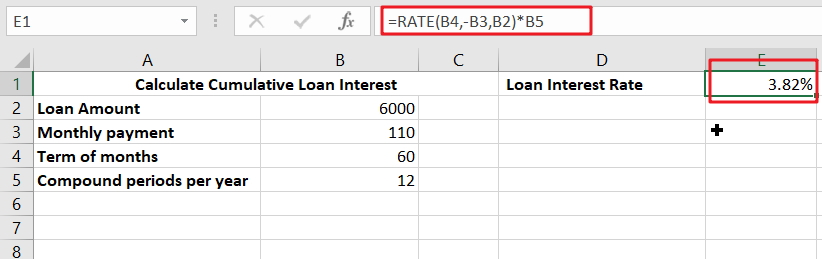# Calculate Interest Rate for Loan

The interest rate is the fee charged by a lender to a borrower and is expressed as a percentage of the principal—the lent amount. The interest rate on a loan is often expressed as an annual percentage rate, abbreviated as APR (APR).

Additionally, an interest rate may be applied to funds earned in a bank or credit union via a savings account or certificate of deposit (CD). The annual percentage yield (APY) on these bank accounts refers to the interest generated. In this post we will check how to calculate interest rate for loan.## Borrower’s Debt Cost

While interest rates indicate the lender’s interest revenue, they represent the borrower’s cost of debt. Businesses compare the cost of borrowing versus the cost of equity, such as dividend payments, to identify the least costly source of financing. Given that the majority of businesses raise capital via debt or equity financing, the cost of capital is examined in order to establish the best capital structure.

## APR vs. APY

Consumer loan interest rates are often expressed as an annual percentage rate (APR). This is the rate of return on investment that lenders need in exchange for the capacity to borrow money. For instance, the annual percentage rate (APR) on credit cards is mentioned. In the example above, 4% represents the annual percentage rate on the mortgage or borrower. The annual percentage rate does not take compounded interest into account.

The annual percentage yield (APY) is the interest rate received on a savings account or certificate of deposit (CD) at a bank or credit union. This interest rate is compounded.

## What is the purpose of the RATE function?

The RATE function is a financial function for interest rate excel that is used to determine the interest rate paid on a loan or the rate of return required to achieve a certain return on an investment over a particular time period.

The RATE function is important for financial analysts since it can be used to determine the interest rate on zero coupon bonds.

`=RATE(nper, pmt, pv, [fv], [type], [guess])`

The RATE function uses the following arguments:

• Nper (required argument) – The total number of periods (months, quarters, years, etc.) over which the loan or investment is to be paid.
• Pmt (required argument) – This is the period-by-period payment. This figure must remain constant during the term of the loan. Principal and interest are included in the monthly payment, but no extra fees or taxes are included. If pmt is not specified, fv must be specified.
• PV (required argument) – The present value of all future payments; the present value of all future payments.
• FV (optional argument) – This is the investment’s target future value. This is the value we want to achieve when the final payment is paid. If we omit fv, it is considered to be zero (the future value of a loan, for example), and a pmt argument must be included in its place.
• Type (optional argument) – Specifies how the formula will handle payment due dates. If no kind is specified or a value of 0 is used, payments are due at the end of the month. If 1 is entered, payments are due at the start of the period.
• Estimation (optional argument) – Our best guess for the appropriate interest rate. This establishes a starting point for the RATE function, allowing it to converge on an answer more quickly than 20 iterations.

### Related Functions

• Excel RATE function
The Excel RATE function returns the interest rate per payment period of an annuity.The syntax of the RATE function is as below:=RATE(nper, pmt,pv,[fv],[type],[guess])….